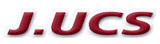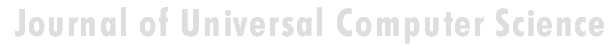Search
 Submission Procedure share: |available in:   PDF (214 kB) PS (166 kB)

get:
 Similar Docs BibTeX Write a comment
get:

DOI:   10.3217/jucs-012-06-0725

### Improving Deterministic and Randomized Exponential-Time Algorithms for the Satisfiability, the Colorability, and the Domatic Number Problem

Tobias Riege (Heinrich-Heine-University Düsseldorf, Germany)

Jörg Rothe (Heinrich-Heine-University Düsseldorf, Germany)

Abstract: NP-complete problems cannot have efficient algorithms unless P = NP. Due to their importance in practice, however, it is useful to improve the known exponential-time algorithms for NP-complete problems. We survey some of the recent results on such improved exponential-time algorithms for the NP-complete problems satisfiability, graph colorability, and the domatic number problem. The deterministic time bounds are compared with the corresponding time bounds of randomized algorithms, which often run faster but only at the cost of having a certain error probability.

Keywords: deterministic algorithms, exact computation, exponential-time algorithms, randomized algorithms

Categories: F.1.2, F.1.3, F.2.2, F.2.3